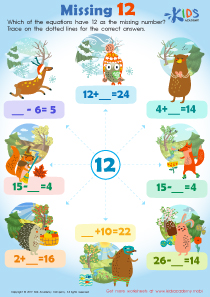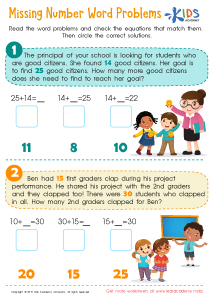# Math Lesson - Enrichment, Grade 1

• ### Activity 1 / Missing 12 Worksheet• ### Activity 2 / Missing Number Word Problems WorksheetWord problems are mathematical problems that come in the form of texts. When your child sees a word problem, they might be confused at first. The key to solving word problems is first reading and understanding them, and then translating the text into an equation. Read the word problems in this worksheet to your students. Help them translate the sentences into a mathematical equation, and then check the equations that match the word problems. Then, circle the correct solutions.

?